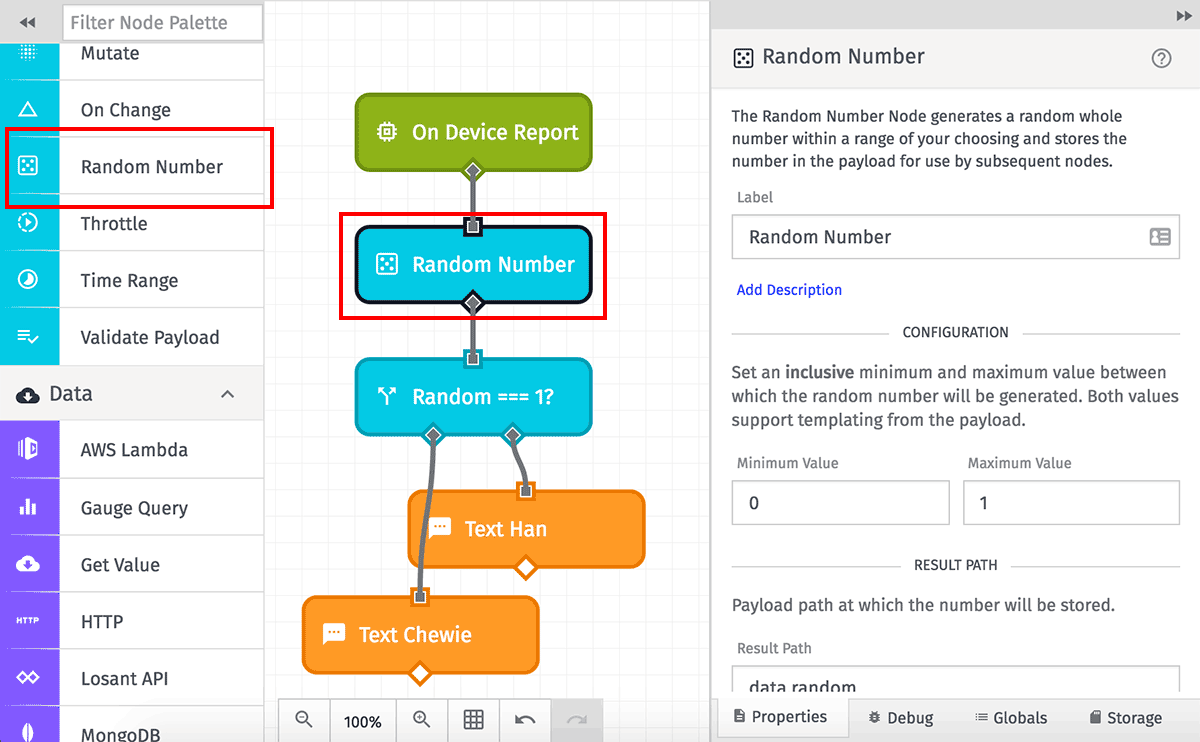# Random Number Node

The Random Number Node generates a random integer within a range of your choosing.## Configuration

The node takes three parameters, all of which are required.

Minimum Value and Maximum Value are each a number or a string template resolving to a numerical value on your payload. The randomly generated number is inclusive of the defined minimum and maximum values; for example, if `0` is set as the minimum value, then `0` is a possible output from the node.

The Result Path is a payload path stipulating where on the payload the resulting number should be stored.

## Node Failure Cases

If the node fails to generate a random number, the result stored on the payload at the result path will be `null`. There are a few of cases where this could occur, most likely when using a string template to reference a value within the payload:

• The minimum or maximum value is not a number. The node attempts to type the value to a number (for example, a string of `"1"` will type to the number `1`) but if that fails, the number generator will return `null`.
• The minimum value is greater than the maximum value. It is, of course, impossible to find a random number in that case.
• There are no integers between the minimum and maximum values. For example, if a minimum value of `1.3` and a maximum value of `1.9` are passed, the node will return `null` because there is no possible random integer between the two values.